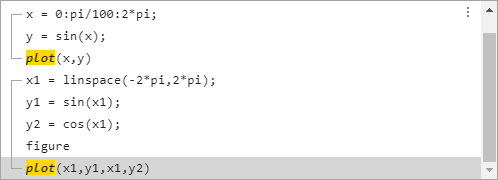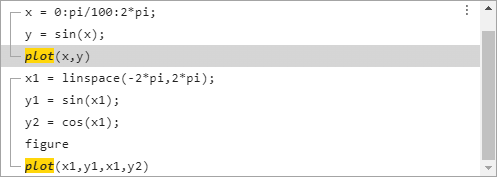# 命令历史记录

## 说明

MATLAB 会将命令行窗口中运行的语句保存到历史记录文件 `History.xml` 中。这些语句包括您使用工具（例如编辑器、命令历史记录窗口和帮助浏览器）中上下文菜单上的执行所选内容选项运行的语句。默认情况下，MATLAB 会在每个语句后自动保存命令历史记录文件。历史记录文件并不包括在 MATLAB 中执行的每项操作。例如，在变量编辑器中对值所做的更改并不包括在命令历史记录窗口中。所有条目均会保留，直到将其删除，或者历史记录文件中的语句数超过了“命令历史记录”预设项中指定的语句保存数为止。当达到指定的范围值时，MATLAB 将自动删除最早的条目。默认情况下，命令历史记录窗口可保存 25,000 条语句。

• 按向上箭头键 (↑)，直到所需的语句显示在提示符处，然后按 Enter

• 在命令历史记录窗口中双击一个或多个条目。

• 在命令历史记录窗口中选择一个条目，然后按 Enter

• 从上下文菜单中选择复制。将所选内容粘贴到编辑器或任何应用程序中打开的文件中。

• 将所选内容从命令历史记录窗口拖动到打开的文件或其他应用程序。

• 点击选择项左侧的“另存为收藏命令”按钮。MATLAB 将在默认收藏命令类别中创建一个新的收藏命令。

• 右键点击并选择创建收藏项。将打开“收藏命令编辑器”对话框，所选条目已添加到代码字段中。配置所需的字段并点击

## 示例

```x = 0:pi/100:2*pi; y = sin(x); plot(x,y) x1 = linspace(-2*pi,2*pi); y1 = sin(x1); y2 = cos(x1); figure plot(x1,y1,x1,y2)```## 编程用途

`commandhistory` 打开 MATLAB 命令历史记录窗口（如果其已关闭），以及选择命令历史记录窗口（如果其已打开）。